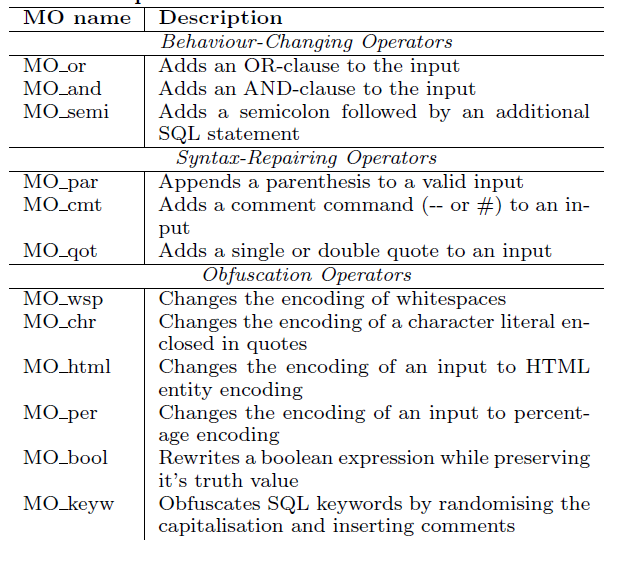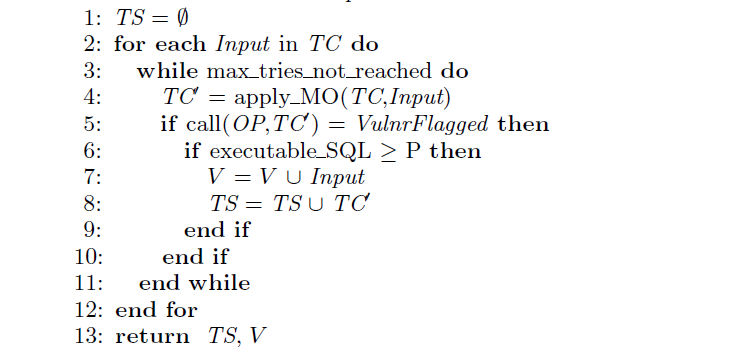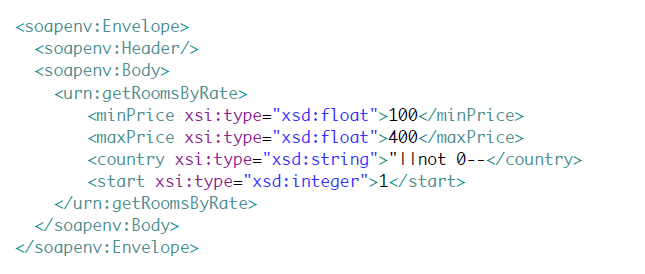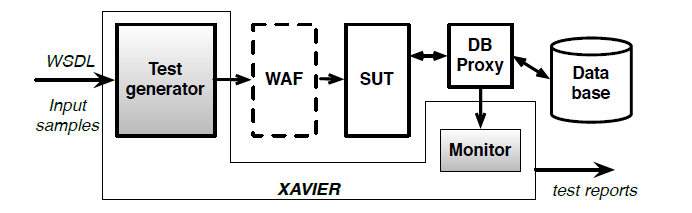# SQL注入漏洞的自动化测试：输入数据变异法(半机翻有删增)

## 摘要

Web服务越来越多地应用于各种领域，从nance和e-government到社交媒体。由于它们是基于Web技术构建的，因此它们也遭受了前所未有的攻击和渗透。在这些攻击中，那些针对SQL注入漏洞的攻击在过去几年一直排名第一。在上线Web服务之前进行测试以检测此类漏洞至关重要。我们在本文中提出了一种自动化测试方法，即μ4SQLi，以及它的基础变异算子集。μ4SQLi可以产生有效的输入，从而导致可执行和有害的SQL语句。可执行性是关键，否则不能利用注入漏洞。我们的评估表明，该方法对于检测SQL注入漏洞并产生绕过现实世界中常见配置的应用程序防火墙是有效的。

## 背景和相关工作

### 背景

$sql = "Select * From hotelList where country ='";$sql = $sql .$country;
$sql =$sql . '"';
$result = mysql_query($sql) or die(mysql_error());


SQLi是一种攻击技术，攻击者将恶意SQL代码片段注入这些输入参数。当输入参数直接在SQL语句中使用而没有经过适当的验证或消毒时，这种攻击是可能的。攻击者可能以改变生成的SQL语句行为的方式构造输入值，使攻击者能够对数据库执行操作，而不是应用程序开发人员所希望的操作。这些操作可能导致敏感数据暴露，未经授权插入或更改数据，丢失数据，甚至控制数据库服务器。

Select * From hotelList where country='' or 1 = 1 --'


Web服务是面向服务的体系结构的基本模块，它提供了在Web上轻松访问和交换信息的工具。每个Web服务都提供一组可由客户端调用的操作。操作类似于传统编程语言中的方法，它具有一组输入参数并返回结构化输出。 Web服务的接口和功能通常由公共可用的Web服务描述语言（WSDL）文件描述。

### 相关工作

(1)我们针对需要不同变异算子和测试生成技术的SQL注入漏洞; (2)在SQL漏洞的情况下，失败的可观察性比寻找崩溃更具挑战性;

## 方法

### 变异算子#### 行为改变

• 向输入添加OR子句
• 在输入中添加AND子句
• 添加分号后跟另外的SQL语句

##### 运算符：MO_or

"SELECT * FROM table WHERE id = "+ input


SELECT * FROM table WHERE id = 1 OR 1 = 1

##### 运算符：MO_and

"SELECT * FROM table WHERE id ="+ input


SELECT * FROM table WHERE id = 1 AND 1 = 2


##### 运算符：MO_semi

"SELECT * FROM users WHERE id = "+ input


SELECT * FROM users WHERE id = 1; SELECT waitfor(5) FROM dual


#### 语法修复

• 将括号附加到有效输入
• 向输入添加注释命令（ - 或＃）
• 为输入添加单引号或双引号

##### 运算符：MO_par

"SELECT * FROM table WHERE character = CHR("+input+")"


SELECT * FROM table WHERE character = CHR(67) OR 1 = 1 -）

##### 运算符：MO_cmt

"SELECT * FROM table WHERE character = CHR("+input+")"


SELECT * FROM table WHERE character = CHR（67）OR 1 = 1 ）


SELECT * FROM table WHERE character = CHR(67) OR 1 = 1＃)


##### 运算符：MO_qot

"SELECT * FROM table WHERE name ='"+input+"'"


SELECT * FROM table WHERE name ='Smith OR 1 = 1'）


SELECT * FROM table WHERE name ='Smith' OR 1 = 1＃）


#### 混淆运算符

• 更改空格的编码
• 更改用引号括起来的字符文字的编码
• 将输入的编码更改为HTML实体编码
• 将输入的编码更改为百分比编码
• 重写布尔表达式，同时保留它的真值
• 通过随机化大写和插入注释来混淆SQL关键字

##### 运算符：MO_wsp

"SELECT * FROM table WHERE id ="+ input


SELECT * FROM table WHERE id = 1 + OR + 1 = 1

##### 运算符：MO_chr

• 短二进制表示，例如，’a’被替换为b’1100001’。
• 长二进制表示，例如，’a’被替换为二进制’1100001’。
• Unicode表示，例如，’a’被替换为n’a’。
• 十六进制表示，例如，’a’被替换为x’61’。

"SELECT * FROM table WHERE id ="+ input


SELECT * FROM table WHERE id = 1 OR'a'= x'61'。

##### 运算符：MO_html

(a)形式为＆#N的数字字符引用，其中N是十进制或十六进制表示中使用的字符集中字符的代码位置; (b)形式为＆SymbolicName的字符实体引用。例如，“是单引号字符（’）的编码。

"SELECT * FROM table WHERE id =" + input


SELECT * FROM table WHERE id = 1 OR &quot;a&quot; = &quot;a&quot;。

##### 运算符：MO_per

"SELECT * FROM table WHERE id ="+ input


SELECT table WHERE id = 1 OR％20'a'='a'

##### 运算符：MO_bool

"SELECT * FROM table WHERE id ="+ input


SELECT * FROM table WHERE id = 1 OR not false = !! 1

##### 运算符：MO keyw

"SELECT * FROM table WHERE id ="+input


SELECT * FROM table WHERE id = 1 || 1 = 1。


### 测试生成

TC：测试用例：ArrayOf(input)
OP：要测试的Web服务操作输出
TS：针对SQLi漏洞的测试套件
V：易受攻击的输入集### 工具## 结论

(1)生成具有修改服务行为的高可能性的输入，
(2)纠正输入以消除由于突变导致的可能的语法错误
(3)混淆攻击以增加他们通过防火墙进行攻击的机会。

## 原文链接

Automated Testing for SQL Injection Vulnerabilities: An Input Mutation Approach